# An Embedded Domain Specific Language for NXCALS and PM Queries

Dear SWAN Users,

I am writing to share with you a package developed for simplifying query and processing of signals from Post Mortem, CALS, and NXCALS databases. The package was created as a part of the Signal Monitoring project I am involved in. The goal of this entry is to share the package with other users as some of you may find it useful in daily work.

The entry is an excerpt from API documentation also available as a SWAN notebook.

# 1. Logging Database Overview

Naturally, one should use a general purpose browser for ad-hoc queries and exploration for signal hierarchy:

In order to programatically query PM, CALS, or NXCALS database, a dedicated API along with parameters is required. An overview of databases from query perspective is summarised in the table below.

PM PM CALS CALS* NXCALS NXCALS
query type event signal signal feature signal feature
input system, source, className system, source, className, signal signal signal, features system, (device, property), signal system, (device, property), signal, features
time definition time range timestamp time range time range time range time range
time unit ns ns s s ns ns
return type json json dict of list dict of list spark dataframe spark dataframe
execution time fast fast can be slow fast slow fast
execution type serial serial serial ? serial parallel
use simple simple simple simple simple requires good knowledge of pyspark

*The pytimber package provides an access to feature queries (for more details, please consult https://github.com/rdemaria/pytimber). Since CALS is about to be discontinued, we decided not to support this query in favor of NXCALS.

The native API for each database is available at:

# 2. Use of Native API to Query Signals in Python

• Post Mortem REST API
``````import pandas as pd
import requests
import json

response = requests.get('http://pm-api-pro/v2/pmdata/signal?system=FGC&className=51_self_pmd&source=RPTE.UA47.RB.A45&timestampInNanos=1426220469520000000&signal=STATUS.I_MEAS')

name = json_response['content']['namesAndValues']['name']
time = json_response['content']['namesAndValues']['value']
value = json_response['content']['namesAndValues']['value']

pd.DataFrame(value, time, [name]).plot()
``````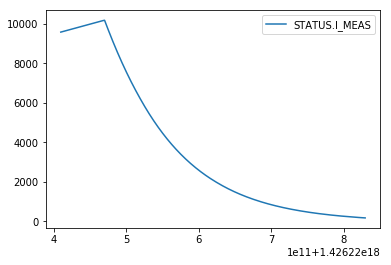Note that for other systems the structure of returned json file may differ and requires dedicated handling

• CALS pytimber API
``````import pandas as pd
import pytimber
ldb = pytimber.LoggingDB()

i_meas = ldb.get("RPTE.UA47.RB.A45:I_MEAS", '2015-03-13 05:20:49.4910002', '2015-03-13 05:22:49.4910002')
pd.DataFrame(i_meas.get("RPTE.UA47.RB.A45:I_MEAS"), i_meas.get("RPTE.UA47.RB.A45:I_MEAS"), ["RPTE.UA47.RB.A45:I_MEAS"]).plot()
``````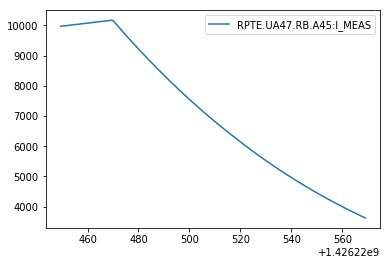• NXCALS API
``````from cern.nxcals.pyquery.builders import *
import pandas as pd

i_meas_df = DevicePropertyQuery.builder(spark).system('CMW') \
.startTime(pd.Timestamp('2015-03-13T04:20:59.491000000').to_datetime64())\
.endTime(pd.Timestamp('2015-03-13T04:22:49.491000000')) \
.entity().device('RPTE.UA47.RB.A45').property('SUB') \
.buildDataset().select('acqStamp', 'I_MEAS').dropna().sort("acqStamp").toPandas()

i_meas_df.set_index(i_meas_df['acqStamp'], inplace=True)
i_meas_df.drop(columns='acqStamp', inplace=True)
i_meas_df.plot()
``````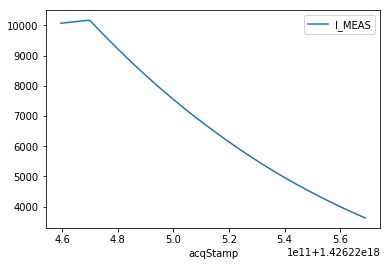Note that signal query with NXCALS is quite time consuming.

# 3. pyeDSL - Embedded Domain Specific Language in Python

Use of the native API in larger analyses requires a lot of code and is difficult to generalise and introduce a structure for repeatable queries. Natural languages have certain structure .

Language Word order Example
English: {Subject}.{Verb}.{Object}: John ate cake
Japanese: {Subject}.{Object}.{Verb}: John-ga keiki-o tabeta
- - John cake ate

One can enforce syntactical order in code:

• Domain Specific Language – new language, requires a parser
• Embedded Domain Specific Language – extends existing language

Furthermore, an eDSL could be implemented following the Fluent interface approach . The use of an eDSL for signal query and processing is not a new concept as there exists already an eDSL in Java used to automatically check signals during Hardware Commisionning campaigns of the LHC .

 K. Gulordava, Word order variation and dependency length minimisation: a cross-linguistic computational approach, PhD thesis, UniGe,
 https://en.wikipedia.org/wiki/Fluent_interface
 M. Audrain, et al. - Using a Java Embedded Domain-Specific Language for LHC Test Analysis, ICALEPCS2013, San Francisco, CA, USA

## 3.1. QueryBuilder

We propose a python embedded Domain Specific Language (pyeDSL) for building queries:

• General purpose query
{DB}.{DURATION}.{QUERY_PARAMETERS}.{QUERY}

e.g.

``````df = QueryBuilder().{with_pm()/with_cals()/with_nxcals()}.with_duration().with_query_parameters()\
.signal_query().dfs
df = QueryBuilder().{with_pm()/with_cals()/with_nxcals()}.with_timestamp().with_query_parameters()\
.signal_query().dfs
``````
• Circuit-oriented query to generalize query across and within circuit types

e.g.

``````df = QueryBuilder().{with_pm()/with_cals()/with_nxcals()}.with_duration().with_circuit_type().with_metadata()\
.signal_query().dfs
.signal_query().dfs
``````

With this approach:

• each parameter defined once (validation of input at each stage)
• single local variable is required
• order of operation is fixed
• vector inputs are supported

Furthermore, the pyeDSL provides hints on the order of execution

``````from lhcsmapi.pyedsl.QueryBuilder import QueryBuilder

QueryBuilder()
``````

Set database name using with_pm(), with_cals(ldb), with_nxcals(spark) method.

``````from lhcsmapi.pyedsl.QueryBuilder import QueryBuilder

QueryBuilder().with_nxcals(spark)
``````

Database name properly set to NXCALS. Set time definition: for PM signal query, with_timestamp(),
for PM event query or CALS, NXCALS signal query, with_duration()

``````from lhcsmapi.pyedsl.QueryBuilder import QueryBuilder

QueryBuilder().with_nxcals(spark).with_duration(t_start='2015-03-13 05:20:59.4910002', duration=[(100, 's'), (100, 's')])
``````

Query duration set to t_start=1426220359491000200, t_end=1426220559491000200. Set generic query parameter using with_query_parameters() method, or a circuit signal using with_circuit_type() method.

At the same time it prohibits unsupported operations throwing a meaningful exception.

``````from lhcsmapi.pyedsl.QueryBuilder import QueryBuilder

QueryBuilder().with_duration(t_start='2015-03-13 05:20:59.4910002', duration=[(100, 's'), (100, 's')])
``````

AttributeError: with_duration() is not supported. Set database name using with_pm(), with_cals(ldb), with_nxcals(spark) method.

A sentence constructed this way maintains the differences of query types while providing a common structure.

### 3.1.1. PM Event Query

Allows finding source and timestamp of PM events within a period of time.

``````from lhcsmapi.pyedsl.QueryBuilder import QueryBuilder

source_timestamp_df = QueryBuilder().with_pm() \
.with_duration(t_start='2015-03-13 05:20:59.4910002', duration=[(100, 's'), (100, 's')]) \
.with_query_parameters(system='FGC', className='51_self_pmd', source='RPTE.UA47.RB.A45') \
.event_query().df

source_timestamp_df
``````
source timestamp
0 RPTE.UA47.RB.A45 1426220469520000000

### 3.1.2. PM Signal Query

Queries signal based on a timestamp as well as system, source, className, and signal.

``````from lhcsmapi.pyedsl.QueryBuilder import QueryBuilder

i_meas_df = QueryBuilder().with_pm() \
.with_timestamp(1426220469520000000) \
.with_query_parameters(system='FGC', source='RPTE.UA47.RB.A45', className='51_self_pmd', signal='STATUS.I_MEAS') \
.signal_query().dfs

i_meas_df.plot()
``````### 3.1.3. CALS Signal Query

Queries signal based on a time duration as well as signal.

``````from lhcsmapi.pyedsl.QueryBuilder import QueryBuilder
import pytimber
ldb = pytimber.LoggingDB()

i_meas_df = QueryBuilder().with_cals(ldb) \
.with_duration(t_start='2015-03-13 05:20:59.4910002', duration=[(100, 's'), (100, 's')]) \
.with_query_parameters(signal='RPTE.UA47.RB.A45:I_MEAS') \
.signal_query().dfs

i_meas_df.plot()
``````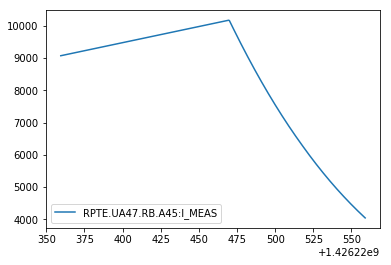### 3.1.4. NXCALS Signal Query

Queries signal based on a time duration as well as system, device, property, and signal.

``````from lhcsmapi.pyedsl.QueryBuilder import QueryBuilder

i_meas_df = QueryBuilder().with_nxcals(spark) \
.with_duration(t_start='2015-03-13 05:20:59.4910002', duration=[(100, 's'), (100, 's')]) \
.with_query_parameters(nxcals_system='CMW', nxcals_device='RPTE.UA47.RB.A45', nxcals_property='SUB', signal='I_MEAS') \
.signal_query().dfs

i_meas_df.plot()
``````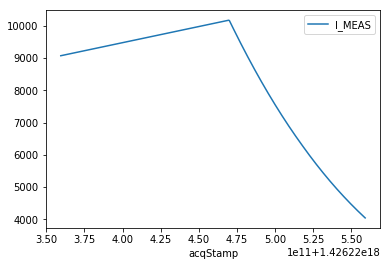Queries signal based on a time duration as well as system and signal.

``````from lhcsmapi.pyedsl.QueryBuilder import QueryBuilder

u_mag_df = QueryBuilder().with_nxcals(spark) \
.with_duration(t_start='2015-03-13 05:20:59.4910002', duration=[(100, 's'), (100, 's')]) \
.with_query_parameters(nxcals_system='WINCCOA', signal='DCBA.15R4.R:U_MAG') \
.signal_query().dfs

u_mag_df.plot()
``````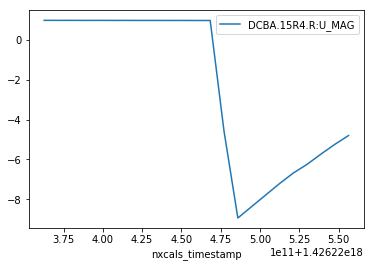### 3.1.5. NXCALS Feature Query

Queries signal features (mean, std, max, min, count) based on a time duration as well as system, device, property, and signal.

``````from lhcsmapi.pyedsl.QueryBuilder import QueryBuilder

i_meas_df = QueryBuilder().with_nxcals(spark) \
.with_duration(t_start=1526898157236000000, t_end=1526903352338000000) \
.with_query_parameters(nxcals_system='CMW', nxcals_device='RPTE.UA23.RB.A12', nxcals_property='SUB', signal='I_MEAS') \
.feature_query(['mean', 'std', 'max', 'min', 'count']).df

i_meas_df
``````
device max min count mean std
0 RPTE.UA23.RB.A12 10978.8 757.18 2498 5374.768659 3315.972856

The same query with native NXCALS API is

``````i_meas_results_ds = DevicePropertyQuery.builder(spark).system('CMW') \
.startTime(t_start_injs).endTime(t_end_sbs) \
.entity().device('RPTE.UA23.RB.A12').property('SUB') \
.buildDataset().select("acqStamp", 'I_MEAS', "device") \
.agg(func.mean('I_MEAS'), func.stddev('I_MEAS'), func.max('I_MEAS'),
func.min('I_MEAS'), func.count('I_MEAS')).collect()

i_meas_results_df = pd.DataFrame(i_meas_results_ds, columns=['I_MEAS_device', 'I_MEAS_class', 'I_MEAS_mean', 'I_MEAS_std', 'I_MEAS_max', 'I_MEAS_min', 'I_MEAS_count']).sort_values(by=['I_MEAS_class'])
i_meas_results_df
``````

Queries signal features (mean, std, max, min, count) based on a time duration as well as system and signal.

``````from lhcsmapi.pyedsl.QueryBuilder import QueryBuilder

u_mag_df = QueryBuilder().with_nxcals(spark) \
.with_duration(t_start=1526898157236000000, t_end=1526903352338000000) \
.with_query_parameters(nxcals_system='WINCCOA', signal='DCBB.8L2.R:U_MAG') \
.feature_query(['mean', 'std', 'max', 'min', 'count']).df

u_mag_df
``````
nxcals_variable_name max min count mean std
0 DCBB.8L2.R:U_MAG 0.002113 -0.975332 51951 -0.196706 0.375195

## 3.2. Polymorphism

pyeDSL allows for processing of lists of signal definitions.

• multiple signal, mutliple sources, multiple systems, multiple className
``````from lhcsmapi.pyedsl.QueryBuilder import QueryBuilder

i_meas_df = QueryBuilder().with_pm() \
.with_timestamp(1426220469520000000) \
.with_query_parameters(system=['FGC', 'FGC'], source=['RPTE.UA47.RB.A45', 'RPTE.UA47.RB.A45'],
className=['51_self_pmd', '51_self_pmd'], signal=['STATUS.I_MEAS', 'STATUS.I_REF']) \
.signal_query().dfs

ax=i_meas_df.plot()
i_meas_df.plot(ax=ax)
``````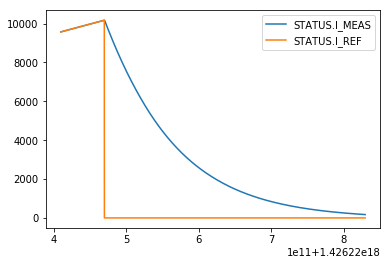• multiple signal, single source, single system, single className
``````from lhcsmapi.pyedsl.QueryBuilder import QueryBuilder

i_meas_df = QueryBuilder().with_pm() \
.with_timestamp(1426220469520000000) \
.with_query_parameters(system='FGC', source='RPTE.UA47.RB.A45',
className='51_self_pmd', signal=['STATUS.I_MEAS', 'STATUS.I_REF']) \
.signal_query().dfs

ax=i_meas_df.plot()
i_meas_df.plot(ax=ax)
``````NXCALS enables calculation of signal features such as min, max, mean, std, count directly on the cluster without the need for costly query of the signal and performing calculation locally. This approach enables parallel computing on the cluster. To this end, a query should contain an element enabling a group by operation. Each group by operation allows for executing computation in parallel.

• Feature query of multiple signals for the same period of time
``````from lhcsmapi.pyedsl.QueryBuilder import QueryBuilder

i_meas_df = QueryBuilder().with_nxcals(spark) \
.with_duration(t_start=1526898157236000000, t_end=1526903352338000000) \
.with_circuit_type('RB') \
.feature_query(['mean', 'std', 'max', 'min', 'count']).df

i_meas_df
``````
device max min count mean std
0 RPTE.UA63.RB.A56 10974.36 756.90 2498 5372.636369 3314.646638
1 RPTE.UA87.RB.A81 10973.21 756.82 2498 5371.596990 3314.230379
2 RPTE.UA83.RB.A78 10967.94 756.47 2498 5369.226934 3312.676833
3 RPTE.UA27.RB.A23 10976.46 757.04 2498 5373.973151 3315.324716
4 RPTE.UA43.RB.A34 10975.16 756.95 2497 5373.559131 3314.074552
5 RPTE.UA67.RB.A67 10978.56 757.18 2498 5374.329179 3315.855302
6 RPTE.UA47.RB.A45 10971.44 756.71 2497 5370.954557 3312.837184
7 RPTE.UA23.RB.A12 10978.80 757.18 2498 5374.768659 3315.972856
• Feature query of multiple signals with the same period of time subdivided into three intervals - group by signal name and interval
``````t_start_injs = 1526898157236000000
t_end_injs = 1526899957236000000
t_start_sbs = 1526901552338000000
t_end_sbs = 1526903352338000000
``````
``````from lhcsmapi.pyedsl.QueryBuilder import QueryBuilder

i_meas_df = QueryBuilder().with_nxcals(spark) \
.with_duration(t_start=t_start_injs, t_end=t_end_sbs) \
.with_circuit_type('RB') \
.signal_query().dfs

ax = i_meas_df.plot(figsize=(10,5), linewidth=5)
ax.axvspan(xmin=t_start_injs, xmax=t_end_injs, facecolor='xkcd:goldenrod')
ax.axvspan(xmin=t_end_injs, xmax=t_start_sbs, facecolor='xkcd:grey')
ax.axvspan(xmin=t_start_sbs, xmax=t_end_sbs, facecolor='xkcd:green')
``````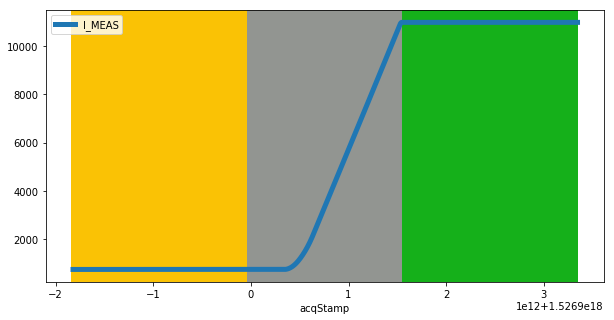Function translate introduces a mapping based on the time column. Here, we consider three subintervals for beam injection, beam acceleration, and stable beams.

As a result, the time column forms a partition and can be executed in parallel.

``````from pyspark.sql.functions import udf
from pyspark.sql.types import IntegerType

def translate(timestamp):
if(timestamp >= t_start_injs and timestamp < t_end_injs):
return 1

if(timestamp >=  t_end_injs and timestamp < t_start_sbs):
return 2

if(timestamp >= t_start_sbs and timestamp <= t_end_sbs):
return 3

return -1

translate_udf = udf(translate, IntegerType())
``````

The translate function should be passed as a function argument

``````i_meas_df = QueryBuilder().with_nxcals(spark) \
.with_duration(t_start=t_start_injs, t_end=t_end_sbs) \
.with_circuit_type('RB') \
.feature_query(['mean', 'std', 'max', 'min', 'count'], function=translate_udf).df

``````
device class max min count mean std
0 RPTE.UA43.RB.A34 1 756.96 756.95 60 756.959833 0.001291
1 RPTE.UA63.RB.A56 3 10974.36 10974.35 60 10974.350167 0.001291
2 RPTE.UA47.RB.A45 3 10971.44 10971.43 60 10971.439667 0.001810
3 RPTE.UA47.RB.A45 2 10970.89 756.71 2377 5346.059971 3193.562789

The same method applied to NXCALS signals based on variable

``````from lhcsmapi.pyedsl.QueryBuilder import QueryBuilder

u_mag_ab_df = QueryBuilder().with_nxcals(spark) \
.with_duration(t_start=t_start_injs, t_end=t_end_sbs) \
.with_circuit_type('RB') \
.with_metadata(circuit_name='RB.A12', system='BUSBAR', signal='U_MAG', wildcard={'BUSBAR': 'DCBB.8L2.R'}) \
.feature_query(['mean', 'std', 'max', 'min', 'count'], function=translate_udf).df

u_mag_ab_df
``````
nxcals_variable_name class max min count mean std
0 DCBB.8L2.R:U_MAG 3 0.002003 -0.034035 18000 -0.001983 0.002882
1 DCBB.8L2.R:U_MAG 1 0.002113 -0.005992 18000 -0.001861 0.002844
2 DCBB.8L2.R:U_MAG 2 0.002041 -0.975332 15951 -0.636315 0.423767

This method can be used together with signal decimation, i.e., taking every nth sample.

For example this can be useful to query QPS board A and B which share the same channel and samples are shifted by 5 so that

• every 10-th sample belongs to board A (or B), decimation=10
``````from lhcsmapi.pyedsl.QueryBuilder import QueryBuilder

u_mag_a_df = QueryBuilder().with_nxcals(spark) \
.with_duration(t_start=t_start_injs, t_end=t_end_sbs) \
.with_circuit_type('RB') \
.with_metadata(circuit_name='RB.A12', system='BUSBAR', signal='U_MAG', wildcard={'BUSBAR': 'DCBB.8L2.R'}) \
.feature_query(['mean', 'std', 'max', 'min', 'count'], function=translate_udf, decimation=10).df

u_mag_a_df
``````
nxcals_variable_name class max min count mean std
0 DCBB.8L2.R:U_MAG 3 0.002003 -0.012705 1800 0.000812 0.000529
1 DCBB.8L2.R:U_MAG 1 0.002113 -0.000463 1800 0.000965 0.000434
2 DCBB.8L2.R:U_MAG 2 0.002041 -0.969405 1595 -0.633601 0.423847
• every 5+10-th sample belongs to board B (or A), decimation=10, shift=5
``````from lhcsmapi.pyedsl.QueryBuilder import QueryBuilder

u_mag_b_df = QueryBuilder().with_nxcals(spark) \
.with_duration(t_start=t_start_injs, t_end=t_end_sbs) \
.with_circuit_type('RB') \
.with_metadata(circuit_name='RB.A12', system='BUSBAR', signal='U_MAG', wildcard={'BUSBAR': 'DCBB.8L2.R'}) \
.feature_query(['mean', 'std', 'max', 'min', 'count'], function=translate_udf, decimation=10, shift=5).df

u_mag_b_df
``````
nxcals_variable_name class max min count mean std
0 DCBB.8L2.R:U_MAG 3 -0.003425 -0.034035 1800 -0.004754 0.000877
1 DCBB.8L2.R:U_MAG 1 -0.003361 -0.005992 1800 -0.004656 0.000441
2 DCBB.8L2.R:U_MAG 2 -0.003052 -0.975332 1595 -0.639233 0.423941
• with polymorphism one can query 1248 busbar at once (in two batches of 624 due to the limit of 1000 signal per query)
``````from lhcsmapi.pyedsl.QueryBuilder import QueryBuilder

u_mag_ab_df = QueryBuilder().with_nxcals(spark) \
.with_duration(t_start=t_start_injs, t_end=t_end_sbs) \
.with_circuit_type('RB') \
.with_metadata(circuit_name='*', system='BUSBAR', signal='U_MAG', wildcard={'BUSBAR': '*'}) \
.feature_query(['mean', 'std', 'max', 'min', 'count'], function=translate_udf).df

``````
nxcals_variable_name class max min count mean std
0 DCBA.22L4.L:U_MAG 1 -0.000167 -0.002102 18000 -0.001110 0.000639
1 DCBA.14L4.R:U_MAG 1 0.001179 -0.000796 18000 0.000171 0.000603
2 DCBA.A28R2.L:U_MAG 3 0.045531 -0.003455 18000 -0.002131 0.001361
3 DCBB.B22L2.R:U_MAG 1 0.000341 -0.003088 18000 -0.001283 0.000557
4 DCBB.11L2.R:U_MAG 3 0.048651 -0.002121 18000 0.001457 0.002587

## 3.4. Processing Raw Signals

Once a signal is queried, one can perform some operations on each of them.
In this case, the order of operations does not matter (but can be checked).

Signal query Signal processing
{DB}.{DURATION}.{QUERY_PARAMETERS}.{QUERY}
.synchronize_time()
.convert_index_to_sec()
.create_col_from_index()
.filter_median()
.remove_values_for_time_less_than()
.remove_initial_offset()
``````from lhcsmapi.pyedsl.QueryBuilder import QueryBuilder

i_meas_df = QueryBuilder().with_nxcals(spark) \
.with_duration(t_start='2015-01-13 16:59:11+01:00', t_end='2015-01-13 17:15:46+01:00') \
.with_circuit_type('RB') \
.signal_query() \
.synchronize_time() \
.convert_index_to_sec().dfs

i_meas_df.plot()
``````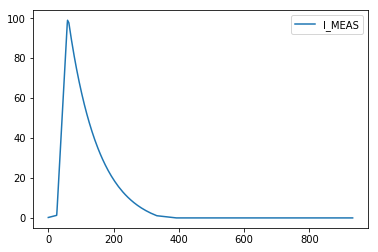2 Likes

Hi,

This is wonderful, would you like to add the notebook (and potentially other notebooks as well) to a SWAN gallery directly accessible from SWAN (via the galleries icon on the top bar)?

This way the interested users would be able to find in SWAN the explanation on how to use the package.

Hi Enric,

absolutely, I’ll add the notebook to the gallery tomorrow. Thank you for a positive response.

Cheers, Michał.